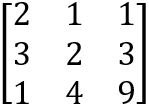### Exam Year

Tribhuvan University

Institute of Science and Technology

2080

Bachelor Level / third-semester / Science

Computer Science and Information Technology( CSC207 )

Numerical Method

Full Marks: 60 + 20 + 20

Pass Marks: 24 + 8 + 8

Time: 3 Hours

Candidates are required to give their answers in their own words as far as practicable.

The figures in the margin indicate full marks.

Section A

Attempt any two questions.

1

How secant methods differs from Newton Rhapson method? Derive the formula for Secant Method. Solve the equation Cosx + 2Sinx – x² = 0 using Secant method. Assume error precision as 0.01. Discuss the drawbacks of the Newton Rhapson method.

2

Define the terms interpolation and extrapolation. Write down the algorithm and program for Newton’s divided difference interpolation.

3

How Gauss Jordan method differs from Gauss Elimination method? Solve the following system of equations using Gauss Jordan method. How can we use Gauss Jordan method to find the inverse of a matrix? Discuss.

2x – y + 4z = 15

2x + 3y – 2z = 4

3x + 2y – 4z = -4

Section B

Attempt any eight questions.

4

Define the terms approximate errot and relative approximate error? Discuss the working of Half Interval method for finding the roots of non-linear equation.

5

Construct Newton’s backward difference table for given data points and approximate the value of f(x) at x=45.

 x 10 20 30 40 50 f(x) 0.985 0.934 0.866 0.766 0.643
6

Fit the quadratic curve through the following data points and estimate the value of f(x) at x=2.

 x 1 3 4 5 6 y 2 7 8 7 5
7

Factorise the following matrix using Cholesky method.8

How can we calculate derivatives of discrete (tabulated) functions? Write down its algorithm.

9

Find the following integral using composite trapezoidal rule for using 2 segments (k=2) and 4 segments (k=4).

24 (x³+2) dx

10

Approximate the solution of y’=3x², y(1)=1 using Taylor’s series method using first four terms. Approximate the value of y(2).

11

Solve the Poisson’s equation ∇²f = xy and f=2 on boundary by assuming square domain 0 ≤ x ≤ 3 and 0 ≤ y ≤ 3 and h=1.

12

Write down the program for solving ordinary differential equation using Heun’s method.